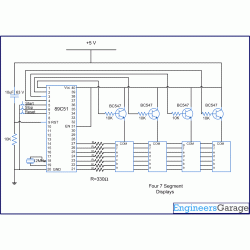# Stopwatch using 8051 microcontroller (AT89C51) PROJECT

Posted by Unknown at 9:39 PMA stopwatch is a timepiece that measures the amount of time between any two occurrences. Usually, at the first occurrence, the stopwatch is started while at the second it is stopped. To use it again, a reset option is also provided with the stopwatch. The total time elapsed can thus be obtained. A stopwatch is very commonly used in racing competitions and other gaming activities. The circuit given here is a digital stopwatch that displays time on four seven segment displays using 8051 microcontroller (AT89C51) .

• The stopwatch keeps the track of time the same way as a simple digital clock does. It is basically an up time counter that starts from 00:00. The control options are provided by means of tactile switches which are active low. This circuit uses three such switches for following operations:
Switch 1 (S1)   :           Start
Switch 2 (S2)   :           Stop
Switch 3 (S3)   :           Reset
Initially when Vcc supply is provided to the circuit, the stopwatch goes in reset mode with 00:00 display state on seven segments. The stopwatch starts running when S1 is pressed. The time is displayed on four seven segments (in common anode configuration) by using the concept of multiplexing. (Also refer Digital Clock) On pressing S2, the stopwatch stops and displays the total time elapsed since the start. It can be taken to reset mode at any instant by using S3.
The seven segments are interfaced to port P2 of the microcontroller AT89C51 through its data pins (a - h). The enable pins are connected to pins 1-4 of port P1 (P1^0 – P1^3). The switches S1-S3 are connected to pins 5-7 of port P1 (P1^4 – P1^6).

•• ```//Program to make a stopwatch

#include
#define msec 1
unsigned int sec1,sec2;
int sec1_1,sec1_2,sec2_1,sec2_2;

unsigned int digi_val={0x40,0xF9,0x24,0x30,0x19,0x12,0x02,0xF8,0x00,0x10};
sbit dig_ctrl_1=P1^0;  // Declare the control pins of seven segments
sbit dig_ctrl_2=P1^1;
sbit dig_ctrl_3=P1^2;
sbit dig_ctrl_4=P1^3;
sbit start_pin = P1^4;  // Start pin to start the watch.
sbit stop_pin = P1^5;  // Stop pin to stop the watch.
sbit reset_pin = P1^6;  // Reset pin to reset the watch.
int s,t;

void mplex_delay(unsigned int time)  // Function to provide a time delay of approximatelty one second using Timer 1
{
int i,j;
for (i=0;i<=time;i++)
for(j=0;j<=50;j++);
}

void digi_out(unsigned int current_num)
{
P2=digi_val[current_num];
mplex_delay(msec);
}

void display(unsigned int dig1,unsigned int dig2)  // Function to display the digits on seven segmnet. For more details refer seven segment multiplexing.
{
sec1_2=dig1%10;
sec1_1=dig1/10;
sec2_2=dig2%10;
sec2_1=dig2/10;
TMOD=0x01;  //Enable Timer 0
TL0=0xFF;
TH0=0xDB;
TR0=1;  // Triger Timer 0
while(TF0==0)
{
dig_ctrl_1 = 1;
dig_ctrl_2 = dig_ctrl_3 = dig_ctrl_4 = 0;
digi_out(sec1_1);
dig_ctrl_2 = 1;
dig_ctrl_1 = dig_ctrl_3 = dig_ctrl_4 = 0;
digi_out(sec1_2);
dig_ctrl_3 = 1;
dig_ctrl_2 = dig_ctrl_1 = dig_ctrl_4 = 0;
digi_out(sec2_1);
dig_ctrl_4 = 1;
dig_ctrl_2 = dig_ctrl_3 = dig_ctrl_1 = 0;
digi_out(sec2_2);
}

TR0=0;
TF0=0;
}

void main()
{
while(1)
{
start:  // Segment to start the stop watch
start_pin = 1;
stop_pin = 1;
reset_pin = 1;
dig_ctrl_1 = 0;
dig_ctrl_2 = 0;
dig_ctrl_3 = 0;
dig_ctrl_4 = 0;
P2 = 0xFF;
s = t = 0;
while(start_pin == 1)// Check if start pin is pressed
{
display(0,0);
}

stopwatch:  // Segment to stop the watch
for (sec1=s;sec1<=99;sec1++)
{
if (stop_pin == 0 )  //Check if stop pin is pressed
break;
for (sec2=t;sec2<=99; sec2++)
{
if (stop_pin == 0 )  //Check if stop pin is pressed
break;
t=0;
display(sec1,sec2);
}
}
stop_pin = 1;
s = sec1;
t = sec2;

while ( start_pin != 0 && reset_pin != 0 )  //Check if start pin or reset pins are not pressed
{
display(sec1,sec2);
}

if (start_pin == 0)  //Check if start pin is pressed
{
goto stopwatch;
}
else
{
if (reset_pin == 0 )  //Check if reset pin is pressed
{
s = t = 0;
goto start;
}
}
}
}```
Tags:

THANKS FOR UR COMMENT ....

## Disclaimer

This blogs is an effort to club the scattered information about engineering and project titles and ideas available in the web. While every effort is made to ensure the accuracy of the information on this site, no liability is accepted for any consequences of using it. Most of the material and information are taken from other blogs and site with the help of search engines. If any posts here are hitting the copyrights of any publishers, kindly mail the details to educations360@gmail.com It will be removed immediately.

## Alexa Rank